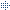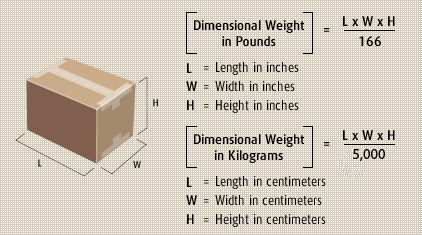Quick Access

Shipment Schedule

Domestic Tariff Rate

Volumetric Calculator

How to fill in your Airway Bill

Standard Terms & Conditions of Carriage

Standard Prohibited Commodities

Volumetric Calculator
 Dimensions in cm: L:   W:   H: Volumetric Weight : ______ KgFormula = Length (cm) x Width (cm) x Height (cm) 6000
Note 1 : About Dimensional Weight

Determining which weight to use in calculating your rate requires that you determine which rule applies. Different rules apply depending on the service selected. Dimensional weight considers density, which is the amount of space a package occupies in relation to its actual weight, to determine billable weight. Calculations of dimensional weight are subject to change without notice.

Note 2 : How to calculate Dimensional WeightFor Example:

• A Customer ships a parcel that is 1kg in actual weight,  the customer's packaging dimensions are 30cm (L) x 25cm (W) x 20cm (H).
• Multiply 30 x 25 x 20 = 15000.
• Then divide 15000 by 6000 to get the Dimensional Weight = 2.5kg.
• The customer will be charged for 2.5kg rather than for actual weight of 1kg.